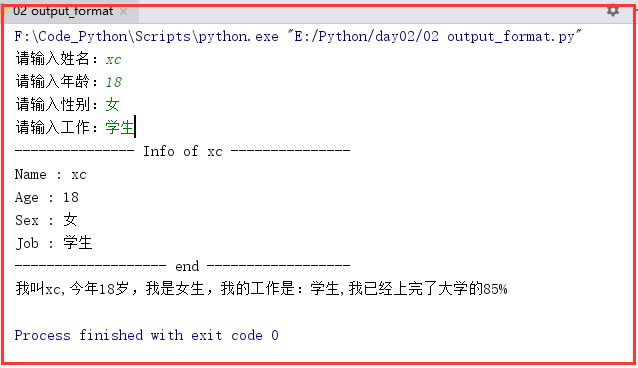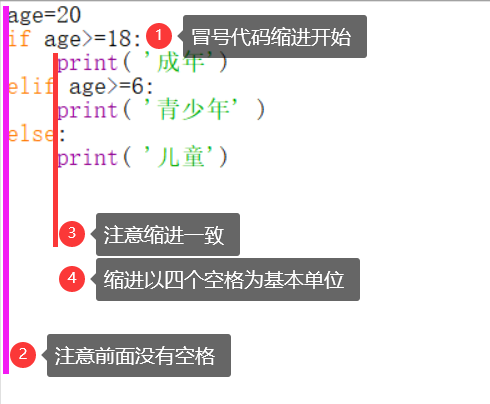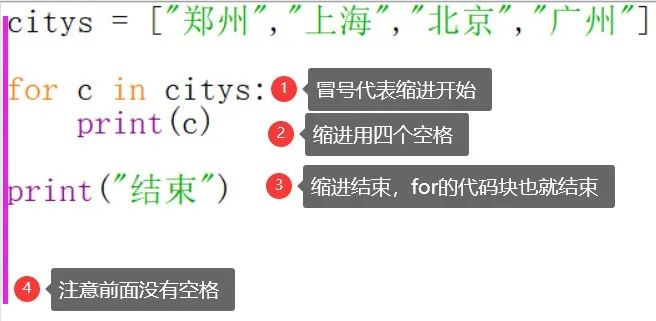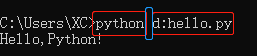# 03python—9个基础常识-python小白入门系列

《python小白入门系列教程》 专栏  • 第03篇

## 1）单行注释：#

#注释内容
print('123')   #123
print('abc')   #abc
print("abc")  #abc

2）多行注释：'''  或  """

(1) 第一种注释方式
'''
code
code
...
'''
(2)第二种注释方式
　"""
code
code
...
"""

## （1）必须由数字，字母，下划线任意组合，且不能数字开头。

（2）不能是python中的关键字。

['and', 'as', 'assert', 'break', 'class', 'continue','def', 'del', 'elif', 'else', 'except', 'exec','finally', 'for', 'from', 'global', 'if', 'import', 'in', 'is', 'lambda', 'not', 'or', 'pass', 'print', 'raise', 'return', 'try', 'while', 'with', 'yield']

（3）变量具有可描述性。
（4）不能是中文。

## 4. 逻辑运算符

（1）or            或者        |

（2）and         并且        &

（3）not            非         ^

## 5. 基础数据类型

（1）数字　　   int　　　　+、-、*、/、%（余数）、**（幂）、//（整除）

（2）字符串　　str　　　　+（拼接）、*（重复）

（3）布尔值　　bool　　　True   False

（4）列表　　　list     　　  增删改查

（5）字典　　　dic　　　　增删改查

（6）元祖　　　tuple　　　增删改查

（7）集合　　   set　　　　增删查

 + 可用于算术加法，还可以用于列表、元组、字符串的连接（但不支持不同类型的

​
* 可用于算术乘法，以及列表、元组、字符串的复制式增加操作。（字典和集合不

​
/ 除法运算 3/2 =1.5
​
// 求整数商 15//4=3 15.0//4=3.0 -15//4=-4 (向下取整)
​
% 求余数
​
** 运算符表示幂乘

x_list = [1, 2, 3]
#创建列表对象
​
x_tuple = (1, 2, 3)
#创建元组对象
​
x_dict = {'a':97, 'b':98, 'c':99}
#创建字典对象
​
x_set = {1, 2, 3}
#创建集合对象
​
print(x_list) #使用下标访问指定位置的元素
2
​
print(x_tuple) #元组也支持使用序号作为下标
2
​
print(x_dict['a']) #字典对象的下标是“键”
97
​
3 in x_set #成员测试
True

s2 = 2
i2 = int(s2)
print(i2,type(i2))  #2 <class 'int'>

s7 = ''
s8 = '非空'
b7 = bool(s7)
b8 = bool(s8)
print(b7,type(b7))     #str为空，则转换为False
print(b8,type(b8))     #str为非空，则转换为True

i1 = 1
s1 = str(i1)
print(s1,type(s1))  #1 <class 'str'>

i3 = 3
i4 = 0
b3 = bool(i3)
b4 = bool(i4)
print(b3,type(b3))  #True <class 'bool'>
print(b4,type(b4))  #False <class 'bool'>

b5 = True
b6 = False
i5 = int(b5)
i6 = int(b6)
print(i5,type(i5))  #1 <class 'int'>
print(i6,type(i6))  #0 <class 'int'>

b1 = Trueb2 = False
s1 = str(b1)
s2 = str(b2)
print(s1,type(s1))  #True <class 'str'>
print(s2,type(s2))  #False <class 'str'>

## 6. 用户交互：input

1）等待输入，
2）将你输入的内容赋值给了前面变量。
3）input出来的数据类型全部是str

## 7.格式化输出：output_format

%　　　  占位符
s　　　　str 字符串
d　　　　digit 数字
n%%　　 表示数字n%

name = input('请输入姓名：')
age = input('请输入年龄：')
sex = input('请输入性别：')
job = input('请输入工作：')
# 为了和占位符%区分开，表示数字5%的时候，需要写成5%%
info = "我叫%s,今年%d岁，我是%s生，我的工作是：%s,我已经上完了大学的85%%" %(name,int(age),sex,job)
msg = '''--------------- Info of %s ---------------
Name : %s
Age : %d
Sex : %s
Job : %s
​
------------------- end ------------------'''%(name,name,int(age),sex,job)
print(msg)
print(info)

运行结果如下：## 8. 缩进

age=20
if age>=18:
print( '成年')
elif age>=6:
print( '青少年' )
else:
print( '儿童')citys = ["郑州","上海","北京","广州"]
​
for c in citys:
print(c)

print("结束")## 9. 运行Python文件

python（空格）文件路径  回车## hello.py 文件需要在对应的文件夹内 这里是 d盘

《python小白入门系列教程》

01-Python安装教程与特色介绍

02-python你应该知道这些IT入门 感谢关注 练习地址：www.520mg.com/it
01-17960
12-152万+09-034万+
08-214282
06-197万+
10-09807
03-272201
08-084万+
09-22159
06-281万+